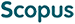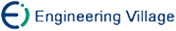谢正卫, 翟 莹, 邓培民, 易 忠. 概率有限状态自动机的代数性质[J]. 计算机研究与发展, 2013, 50(12): 2691-2698.
 引用本文: 谢正卫, 翟 莹, 邓培民, 易 忠. 概率有限状态自动机的代数性质[J]. 计算机研究与发展, 2013, 50(12): 2691-2698.Xie Zhengwei, Zhai Ying, Deng Peimin, Yi Zhong. Algebraic Properties of Probabilistic Finite State Automata[J]. Journal of Computer Research and Development, 2013, 50(12): 2691-2698.
 Citation: Xie Zhengwei, Zhai Ying, Deng Peimin, Yi Zhong. Algebraic Properties of Probabilistic Finite State Automata[J]. Journal of Computer Research and Development, 2013, 50(12): 2691-2698.## Algebraic Properties of Probabilistic Finite State Automata

• 摘要: 利用矩阵、同态、同构、同余等代数工具研究概率有限状态自动机的代数性质.首先定义了输入集上两个字符串同余的概念，并利用概率转移矩阵给出2个字符串同余的一些等价刻画.进而提出概率有限状态自动机同态和同构的概念,并给出了概率有限状态自动机同态定理.证明了2个概率有限状态自动机同构的充要条件是它们的概率转移矩阵可以通过第1种行列初等变换相互转化；同时提出了2个概率有限状态自动机积与和的概念，并得到了积自动机、和自动机的同态关系.最后将模糊自动机中交换的概念引入到概率有限状态自动机中，并利用概率转移矩阵给出了此类自动机交换的一些等价刻画以及和自动机、积自动机交换的充要条件.

Abstract: Probabilistic finite state automata(PFA)is widely used today in a variety of areas in pattern recognition, or in fields to which pattern recognition is linked：computational linguistics, machine learning, time series analysis, circuit testing, computational biology, speech recognition, and machine translation are some of them. In this paper, algebraic properties of PFA are studied by using algebraic tools such as matrices, homomorphisms, isomorphisms, congruences, and so on. Firstly, the concept of congruences of two strings over input alphabet is defined, and some equivalent characterizations of congruences of two strings are given by probabilistic transition matrices.Secondly, notions of homomorphisms and isomorphisms of PFA are introduced, and homomorphism theorem of PFA is shown. It is also proved that two PFA are isomorphic if and only if their probabilistic transition matrices can be transformed into each other by the first kind of elementary transformation of rows and columns. The concept of products and sums of PFA is defined, and the homomorphism relationships between products and sums of PFA are investigated. Finally, the concept of commutativity of fuzzy finite state machines is introduced for PFA, and several equivalent characterizations of commutativity of PFA are obtained as well as the sufficient and necessary conditions of the commutativity of products and sums of PFA by probabilistic transition matrices./下载:  全尺寸图片 幻灯片
• 分享
• 用微信扫码二维码

分享至好友和朋友圈